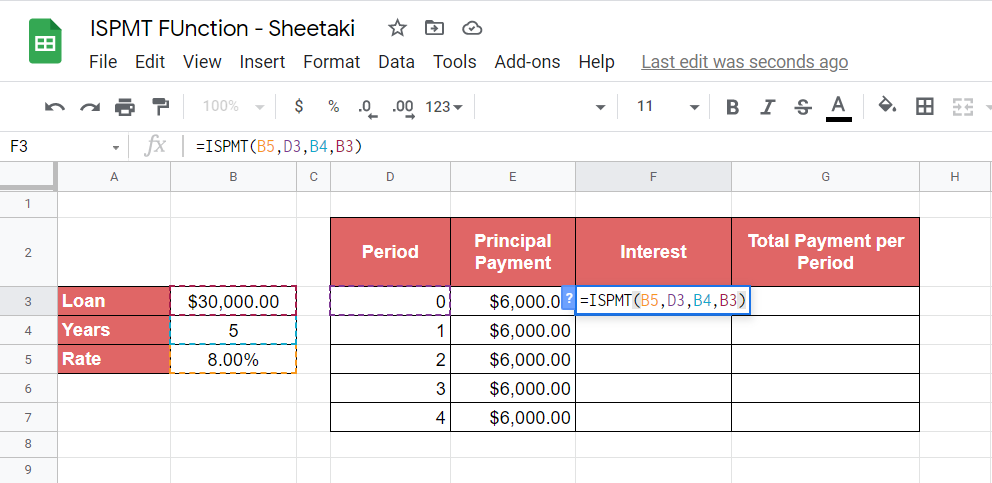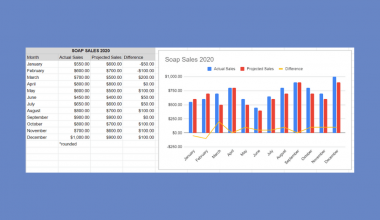# How to Use ISPMT Function in Google Sheets

The ISPMT function in Google Sheets is useful when you want to compute the interest payment for a particular loan or investment period.

Google Sheets simplified most of the complicated and time-consuming accounting operations through its financial functions. One of these functions is ISPMT, which can be utilized to simplify the computation of interests.

With this function, you don’t have to worry about writing complex formulas just so you can keep track of your loans. It’s important to note, however, that this function is especially for even principal payments. If it’s otherwise, you’ll need to use another function called `IPMT`.

Let’s say you’re planning to apply for a 6-year loan from your bank amounting to \$30,000 with a 10% annual interest rate.

If you decide to make even payments for 6 periods, calculating the principal payment for every period should be easy since you need to divide the loan amount by the number of terms, which, in this case, is 6. A simple computation yields you a result of \$5000 per period.

Solving for the principal payments is just basic math, but what about the interest and your actual payment for every term? You’ll have to include different factors such as the loan amount, period, principal payment per period, and interest rate. This makes the computation a lot more confusing, especially if you’re not familiar with accounting jargon.

Thankfully, the ISPMT function simplifies this work for you. Follow along with this article so that you’ll learn how to utilize it in real-world situations efficiently.

## The Anatomy of the ISPMT Function

Writing a function in Google Sheets requires a particular syntax or structure. For ISPMT, this is how we should write it:

=ISPMT(rate, period, number_of_periods, present_value)
All parameters of the ISPMT function are required. Let’s break down the syntax and understand each parameter:
• = just like other functions, the equal sign is the first character we should type in to use ISPMT.
• ISPMT is the function that we’ll need to compute for the interest payment.
• rate is our first parameter. It should contain the interest rate of the loan.
• per should hold the period for which you need to figure out the interest. Keep in mind that ISPMT counts each period beginning with zero. For instance, in a 6-year loan, the period will be counted from 0 to 5.
• number_of_periods parameter defines the required number of payment periods of the loan.
• present_value is the total loan amount.

## A Real Example of Using ISPMT Function

To further explain how the ISPMT function works, let’s take a look at the example below.The data is all about a 5-year, \$15,000 loan with an annual rate of 5%. We have a fixed principal payment of \$3,000 spread across five periods, so we used the ISPMT function to calculate interest for each period. The values of the Interest column are in negative form, signifying that they are outgoing payments.

In all our formulas, the first parameter (rate) holds cell B4 with a value of 5%. For our second parameter (per), you’ll notice the cell reference on each formula depends on the corresponding period. This means that every period has a different computation of interest.

Take the first period for example. We defined cell D3 having a value 0 as the per parameter and it resulted in a \$750 interest.

For each formula, we also indicated 5 (B3) as the number_of_periods and \$15,000 (B2) to be the present_value parameter.

You can have a copy of the example data and experiment with it through the link below.

Once you learn how to compute the interest using ISPMT, you can use it and other functions in Google Sheets to provide you additional information about your loans.

Now, let’s try a simple activity to utilize your knowledge of ISPMT and understand more of its practical applications. Make sure to have a copy of the example spreadsheet as it contains the data for our activity.

## How to Use ISPMT Function in Google Sheets

1. With the copy of the spreadsheet already open, click Sheet2. You should have this data on your screen.Our main objective is to find out the total payment per period of the loan amounting to \$30,000. The formula for this is simple. We just need to add the principal payment and interest of each period. But, to do so, we need to solve for the interest of each payment period first.
2. We’ll start by computing the interest of our first period, which is 0. We need to display it on cell F3, so click the cell to make it active.
3. Type the equal sign ‘=’ followed by ISPMT to start our function. While doing this, you’ll see that an auto-suggest box appears. It’s up to you if you want to just click on the suggestion or finish typing ‘ISPMT(.4. This time, we’ll need to specify the parameters of our function. The values are already provided in the spreadsheet; hence, we can just use their cell references. As you can see, the interest rate for this loan (8%) is located at cell B5. Let’s indicate it on our rate parameter.5. Next, let’s specify the period at which we want to know the interest. People sometimes confuse this parameter with number_of_periods. To clear out any doubt, the period should reflect the current payment period and number_of_periods, as the name implies, the total number of payment periods.
Let’s type in the current period, D3, at our period parameter.6. To complete our ISPMT function, let’s add the remaining cell references to the number_of_periods and present_value parameters. Let’s type in B4, and B3 respectively. Also, don’t forget to close the formula by typing the close parenthesis ‘)’.7. Upon closing the formula, press the Enter key on your keyboard. You should have an output similar to this.8. Remember that the ISPMT function returns a negative value to denote outgoing payment. Our case would require us to convert it to positive since we still need to solve for the total payment per period. Otherwise, our computation would be incorrect.

To fix this, simply add a minus sign (-) right after the equal sign of our formula as shown below and hit the Enter key afterward.This should now be your output.9. Now that we have solved the interest for our first period, we can just use the Autofill feature of Google Sheets to find out the succeeding interests. However, doing this right away may cause errors in your spreadsheet because our formula contains absolute cell references, which Google Sheets cannot detect.
Adding a dollar sign (\$) for all cell references that should remain constant should fix this issue. In our case, the rate, number_of_periods, and present_value are the fixed values, so set them as absolute references. To do so, update your formula to look like this.
=-ISPMT(\$B\$5,D3,\$B\$4,\$B\$3)
After updating the formula, use the Autofill feature to solve for the remaining interests.10. We now have the interests for all payment periods. With this data, we can already compute the total payment. On the Total Payment per Period column, write the formula that adds the Principal Payment and Interest for each period.
You can also copy the formula below and paste it to cell G3 to fast-track your progress. Just use Autofill to fill out the remaining cells.
=E3+F3Great work! Now you know how ISPMT works. Surely you realize by now how much simpler your work will be with this function. You can use it along with other Google Sheets formulas to make your work more efficient.

##### You May Also Like## How to Use YEARFRAC Function in Google Sheets

The YEARFRAC function in Google Sheets is useful if you want to get a decimal value that represents…## How to Use the SUMIF Function Horizontally in Google Sheets

The SUMIF function in Google Sheets is useful to get the sum of cells that meet the provided…## How to Create a Combo Chart in Google Sheets

A combo chart combines visual points of two or more charts to present information in one cohesive and…## How to Use Query With Importrange in Google Sheets

The Query with Importrange in Google Sheets is useful if you want to pull the exact data that…